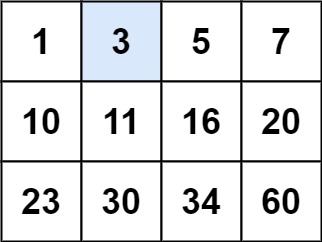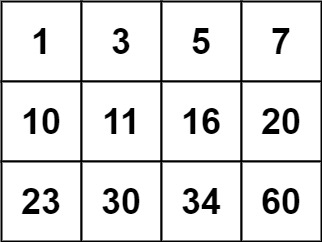## 74. Search a 2D Matrix

Write an efficient algorithm that searches for a value in an `m x n` matrix. This matrix has the following properties:

• Integers in each row are sorted from left to right.
• The first integer of each row is greater than the last integer of the previous row.

Example 1:```Input: matrix = [[1,3,5,7],[10,11,16,20],[23,30,34,60]], target = 3
Output: true
```

Example 2:```Input: matrix = [[1,3,5,7],[10,11,16,20],[23,30,34,60]], target = 13
Output: false
```

Constraints:

• `m == matrix.length`
• `n == matrix[i].length`
• `1 <= m, n <= 100`
• `-104 <= matrix[i][j], target <= 104`

## Rust Solution

``````struct Solution;

impl Solution {
fn search_matrix(matrix: Vec<Vec<i32>>, target: i32) -> bool {
let mut a = vec![];
for row in matrix {
for x in row {
a.push(x);
}
}
a.binary_search(&target).is_ok()
}
}

#[test]
fn test() {
let matrix: Vec<Vec<i32>> = vec_vec_i32![[1, 3, 5, 7], [10, 11, 16, 20], [23, 30, 34, 50]];
let target = 3;
let res = true;
assert_eq!(Solution::search_matrix(matrix, target), res);
}
``````

Having problems with this solution? Click here to submit an issue on github.